Univerza na Primorskem Fakulteta za matematiko, naravoslovje in informacijske tehnologije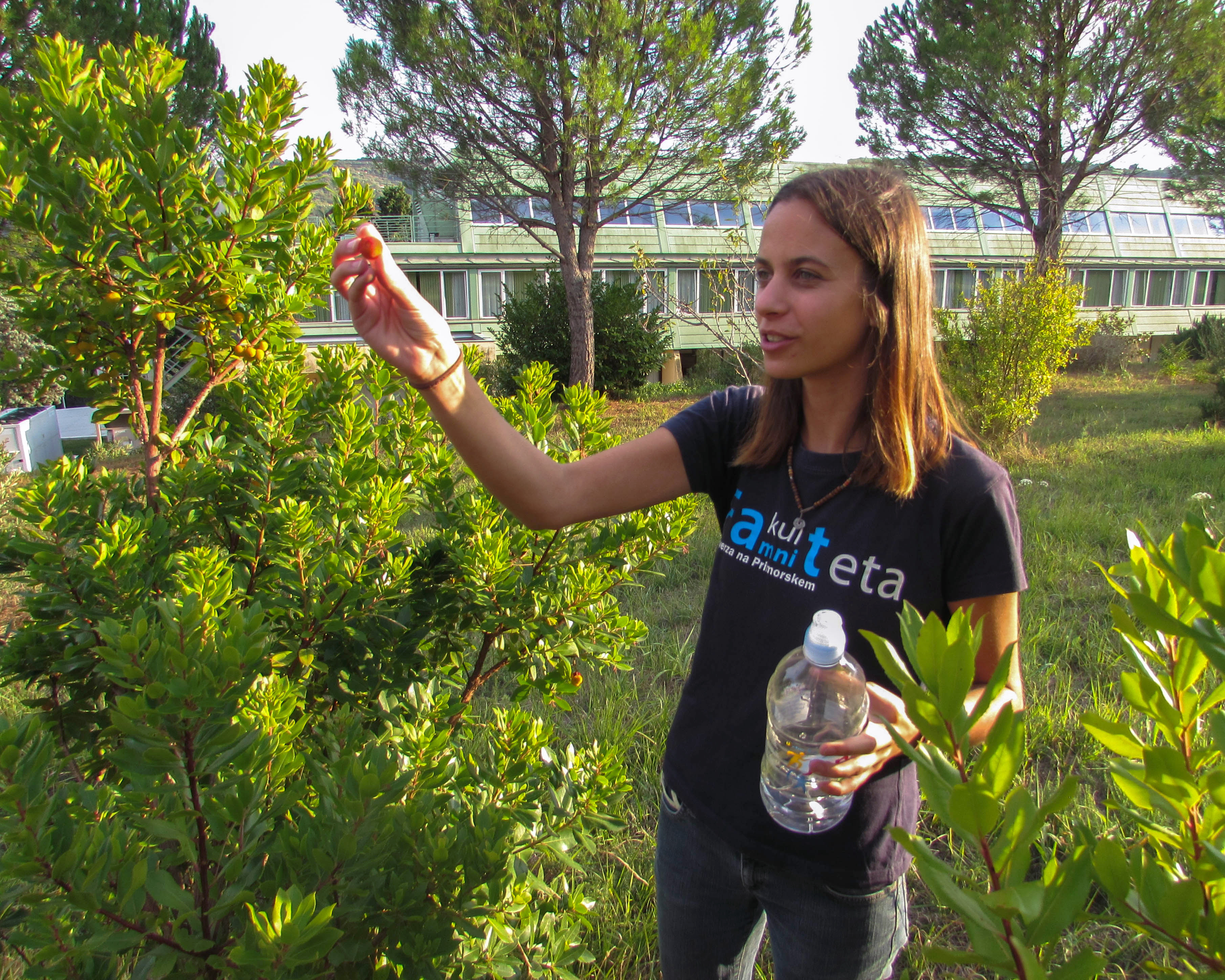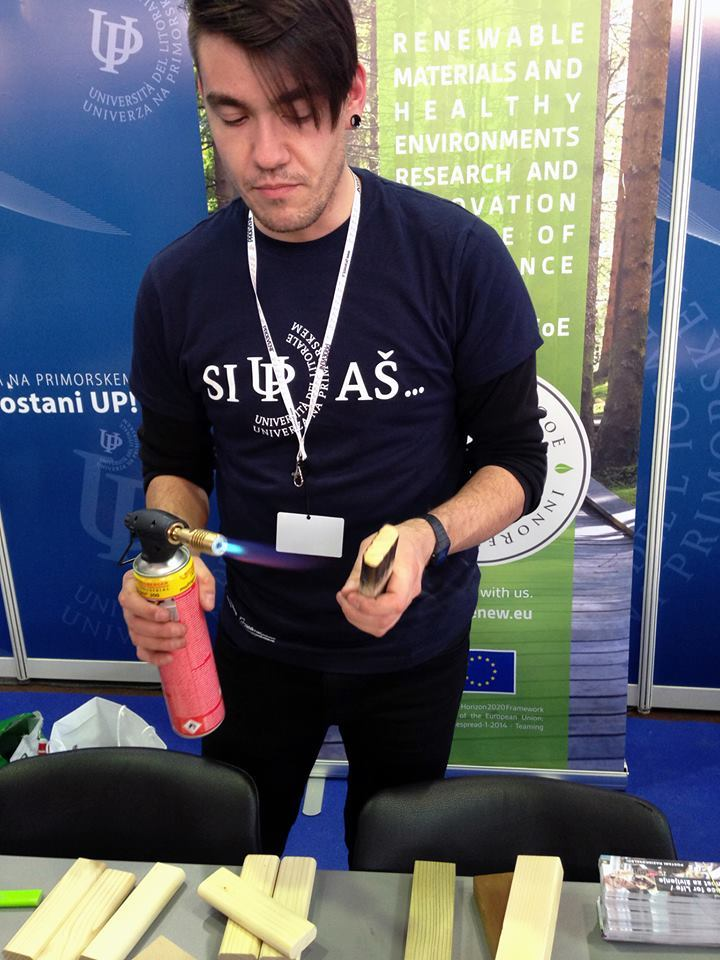SI | EN# Raziskovalni matematični seminar - Arhiv

 2022 2021 2020 2019 2018 2017 2016 2015 2014 2013 2012 2011 2010
 1 2 3 4 5 6 7 8 9 10 11 12
Datum in ura / Date and time: 29.12.14
(10:00-11:00)
Predavalnica / Location: FAMNIT-SEMIN
Predavatelj / Lecturer: prof. dr. Vladimir Gurvich (Rutgers University, USA)
Naslov / Title: On Nash-solvability of chess-like games
Vsebina / Abstract:

In 2003, Boros and Gurvich proved that a chess-likes game has a Nash equilibrium (NE) in pure stationary strategies if (A) the number n of players is at most 2, or (B) the number p of terminals is at most 2 and (C) any infinite play is worse than each terminal for every player. In the talk we strengthen the bound in (B) replacing p <= 2 by p <= 3, provided (C) still holds. On the other hand, we construct an NE-free four-person chess-like game with five terminals, which has a unique cycle, but does not satisfy (C). It remains open whether an NE-free example exists for n = 3, or for 2 < p < 4, or for some n > 3 and p > 4 provided (C) holds.

Joint work with E. Boros, V. Oudalov, and R. Rand.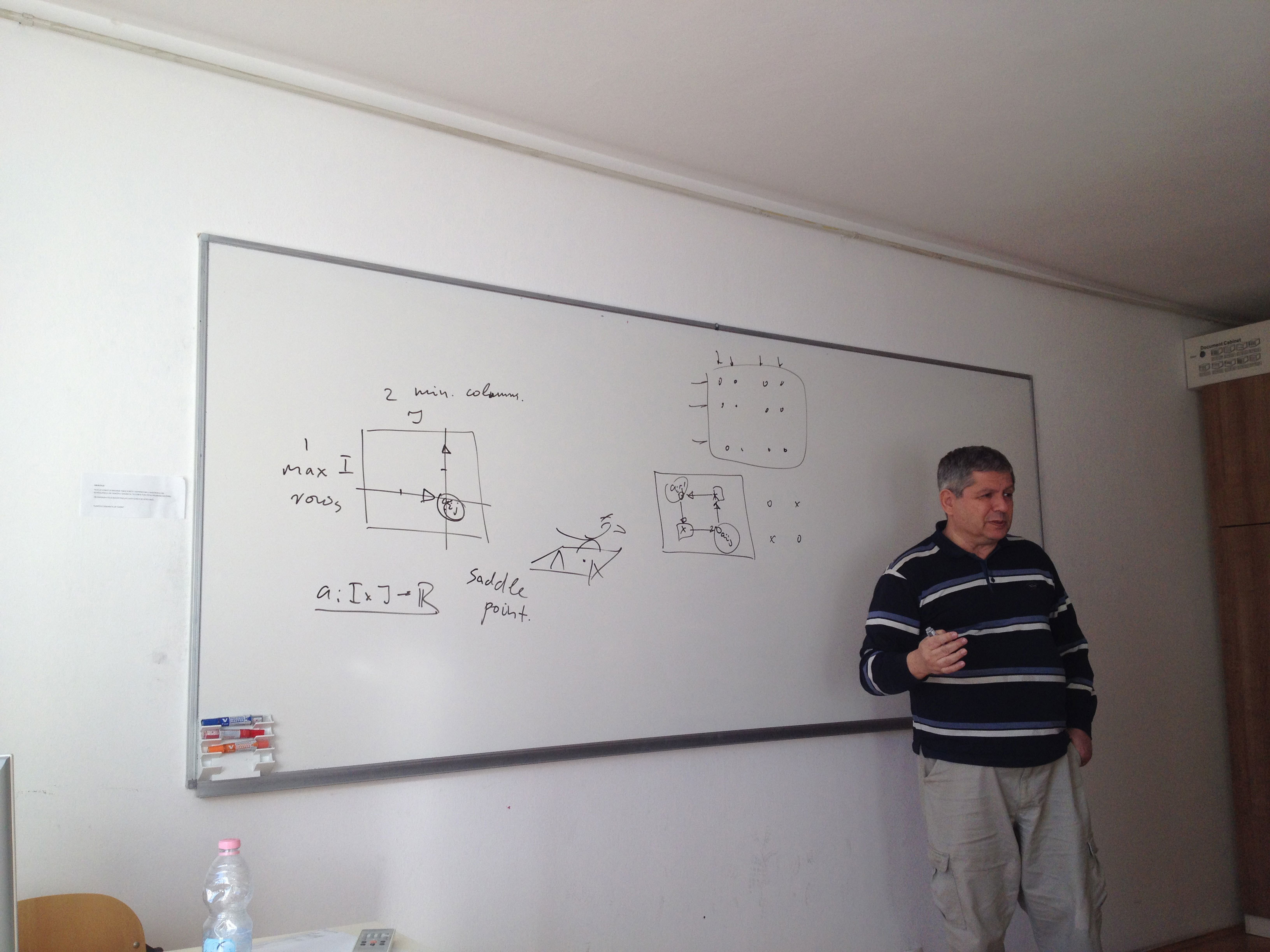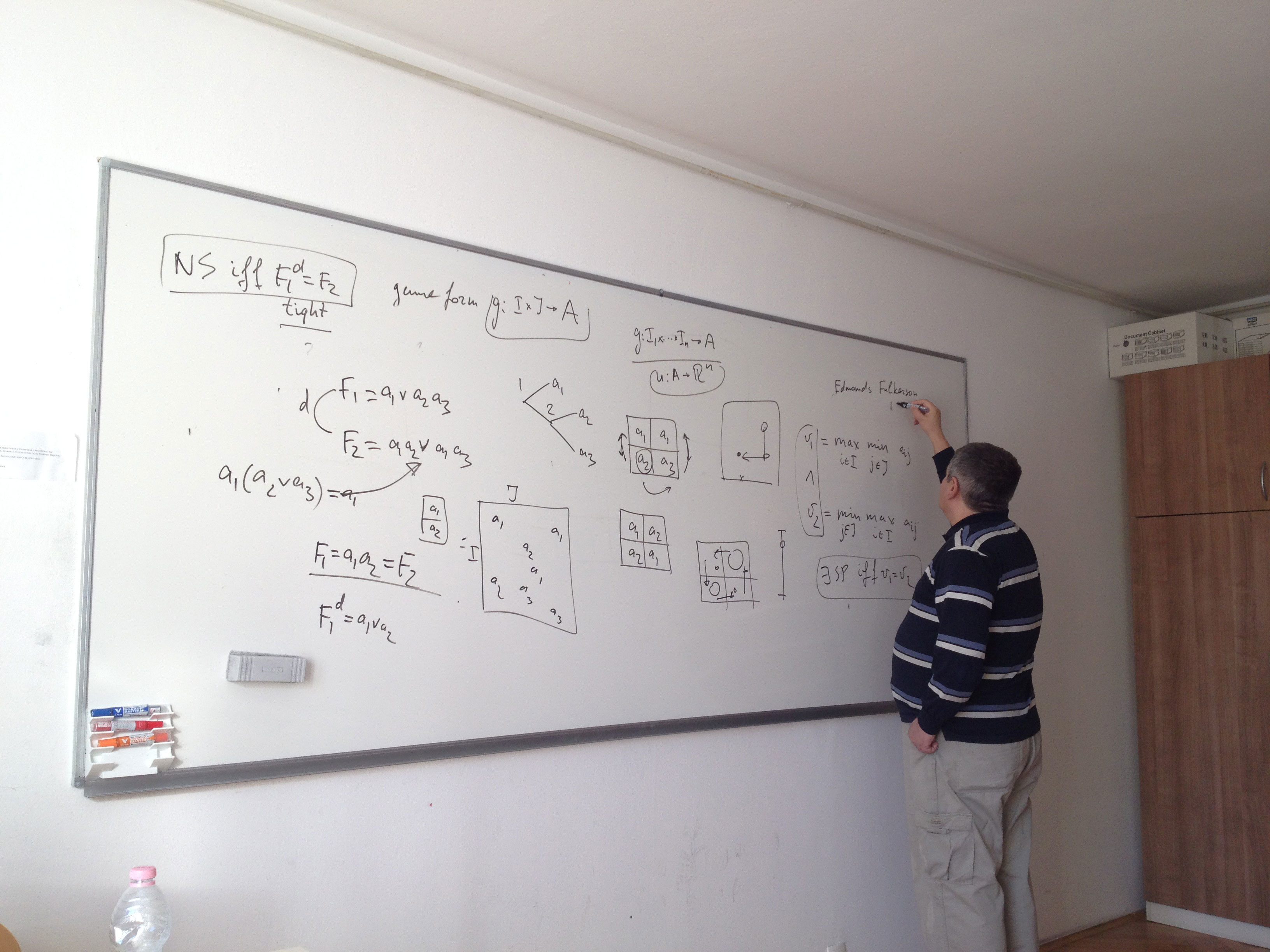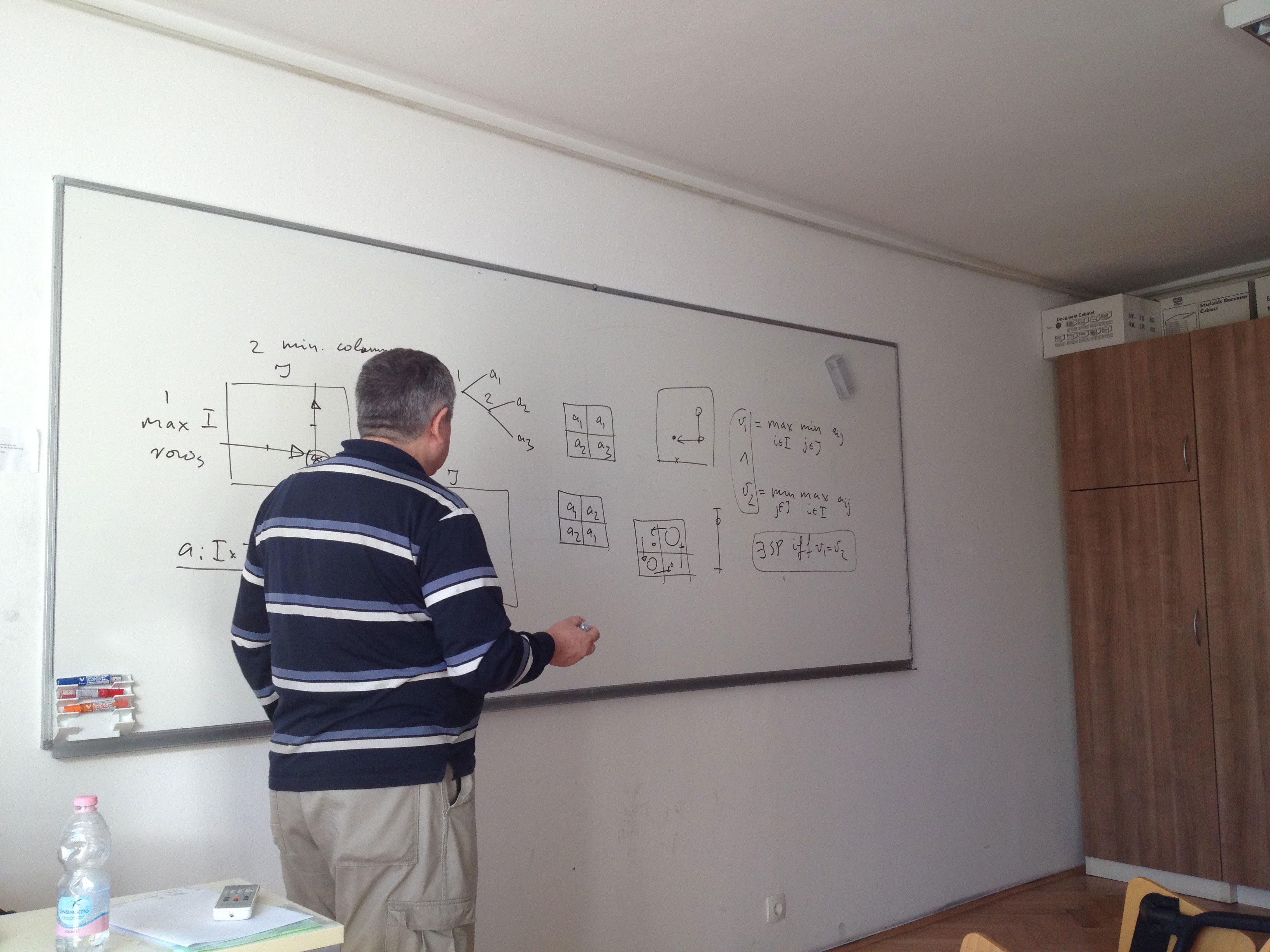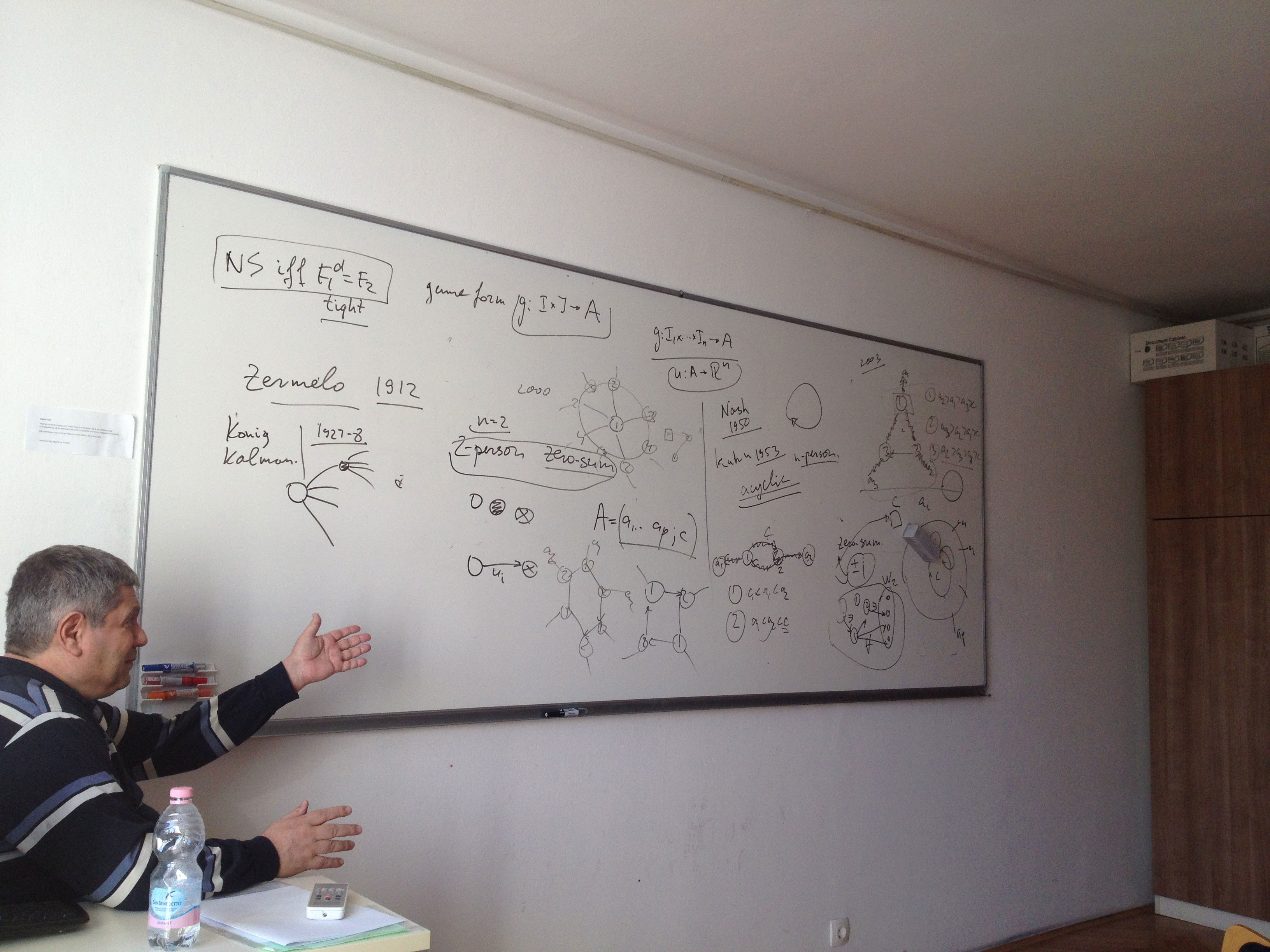http://rutcor.rutgers.edu/pub/rrr/reports2014/09_2014.pdf

http://arxiv.org/pdf/1411.0349.pdf

Datum in ura / Date and time: 22.12.14
(10:00-11:00)
Predavalnica / Location: FAMNIT-SEMIN
Predavatelj / Lecturer: dr. Martin Milanič (UP FAMNIT)
Naslov / Title: Vector connectivity in graphs
Vsebina / Abstract:

Motivated by challenges related to domination, connectivity, and information propagation in social and other networks, we study the Vector Connectivity problem. This problem takes as input a graph G and an integer r(v) for every vertex v of G, and the objective is to find a vertex subset S of minimum cardinality such that every vertex v either belongs to S, or is connected to at least r(v) vertices of S by disjoint paths. If we require each path to be of length exactly 1, we get the well-known vector domination problem, which is a generalization of the dominating set and vertex cover problems. Consequently, the vector connectivity problem becomes NP-hard if an upper bound on the length of the disjoint paths is also supplied as input. Due to the hardness of these domination variants
even on restricted graph classes, like split graphs, Vector Connectivity seems to be a natural problem to study for drawing the boundaries of tractability for this type of problems.

In the talk, we will give an overview of known complexity results for the Vector Connectivity problem. In particular, the problem can be solved in polynomial time on split graphs, in addition to cographs, trees, and block graphs. On the other hand, the problem is NP-hard for planar line graphs and for planar bipartite graphs, APX-hard on general graphs, and can be approximated in polynomial time within a factor of log n + 2 on all n-vertex graphs. Vertex covers and dominating sets in a graph G can be easily characterized as hitting
sets of derived hypergraphs (of G itself, and of the closed neighborhood hypergraph of G, respectively). Using Menger's Theorem, a similar characterization of vector connectivity sets can be derived.

Based on joint works with Endre Boros, Ferdinando Cicalese, Pinar Heggernes, Pim van 't Hof, and Romeo Rizzi.

Datum in ura / Date and time: 15.12.14
(10:00-12:00)
Predavalnica / Location: FAMNIT-MP1
Predavatelj / Lecturer: Alexander Vasilyev
Naslov / Title: On Adriatic Indices, Spectral Properties of Adriatic Matrices and Software for Topological Descriptors

Datum in ura / Date and time: 8.12.14
(11:15-12:15)
Predavalnica / Location: FAMNIT-SEMIN
Predavatelj / Lecturer: Emil Žagar
Naslov / Title: Geometric interpolation by parametric polynomial curves
Vsebina / Abstract:

Interpolation of curves and surfaces is a fundamental problem in computer aided
geometric design (CAGD) and related fields of research. In this talk we shall
focus on interpolation of parametric curves (or data) by parametric polynomial
curves. In contrast to functional case, where a polynomial of degree n can, in
general, interpolate at most n+1 points, planar parametric polynomial curve of
degree n might interpolate as much as 2n points. This kind of interpolation is
known as ’geometric interpolation’. Some results and open problems related to
geometric interpolation will be presented and interpolation of special parametric
objects (such as circular arcs) will be briefly outlined.